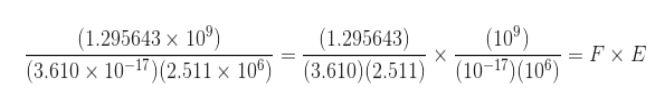# Use scientific notation and laws of exponents to solve. Enter your answer rounded to the number of significant digits inidcated by the given data.  (1.295643 x 109)/((3.610 x 10-17)(2.511 x 106))I did this: (1.295643)/((3.610)(2.511)), times 109-17+6  and I got 0.1429 x 10-2 but the answer guide says that the answer is 0.1429 x 1019. Should I be adding the exponents differently?

Question
53 views

Use scientific notation and laws of exponents to solve. Enter your answer rounded to the number of significant digits inidcated by the given data.

(1.295643 x 109)/((3.610 x 10-17)(2.511 x 106))

I did this:

(1.295643)/((3.610)(2.511)), times 109-17+6  and I got 0.1429 x 10-2 but the answer guide says that the answer is 0.1429 x 1019. Should I be adding the exponents differently?

check_circle

star
star
star
star
star
1 Rating
Step 1

Let's separate the given expression into two parts: the fractional parts F and the exponential part E. Plese see the whiteboard.help_outlineImage Transcriptionclose(100) (1.295643 x 10) (1.295643) (3.610) (2.511) * (10-17)(10= F x £ (3.610 x 10-17)(2.511 x 100) fullscreen
Step 2

Let's handle them one by one. Please see the white board where we have simplified F.

Step 3

Let's now attempt to simplify E. Recall the fomous l...

### Want to see the full answer?

See Solution

#### Want to see this answer and more?

Solutions are written by subject experts who are available 24/7. Questions are typically answered within 1 hour.*

See Solution
*Response times may vary by subject and question.
Tagged in
MathCalculus

### Functions